## ML Aggarwal Class 7 Solutions for ICSE Maths Chapter 2 Fractions and Decimals Ex 2.5

Question 1.
Write the following numbers in the expanded form:
(i) 20.03
(ii) 200.03
(iii) 2.034
Solution: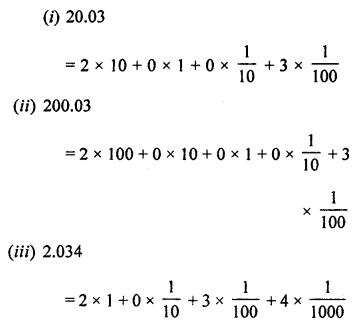Question 2.
Write the place value of digit 2 in the following decimal numbers:
(i) 2.56
(ii) 21.37
(iii) 10.25
(iv) 63.352
Solution:
(i) 2.56, place value of 2 = 2 × 1 = 2
(ii) 21.37, place value of 2 = 2 × 10 = 20
(iii) 10.25, place value of 2 = 2 × $$\frac { 1 }{ 10 }$$ = $$\frac { 2 }{ 10 }$$
(iv) 63.352, place value of 2 = 2 × $$\frac { 1 }{ 1000 }$$ = $$\frac { 2 }{ 1000 }$$

Question 3.
Convert the following decimal numbers to frac ions (in simplest form):
(i) 0.8
(ii) 0.225
(iii) 0. 0092
(iv) 3.025
Solution: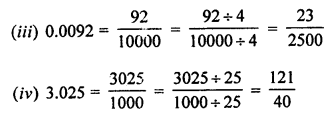Question 4.
Convert the following decimals to mixed fractions:
(i) 5.05
(ii) 63.125
(iii) 17.075
(iv) 317.0006
Solution:Question 5.
Convert the following fractions into decimal numbers:
(i) $$\frac { 3 }{ 5 }$$
(ii) $$\frac { 7 }{ 8 }$$
(iii) 3$$\frac { 5 }{ 16 }$$
(iv) 137$$\frac { 13 }{ 62 }$$
Solution: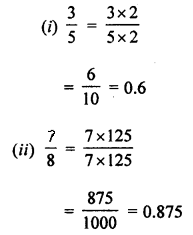Question 6.
Which is greater?
(i) 0.5 or 0.05
(ii) 7 or 0.7
(iii) 2.03 or 2.30
(iv) 0.8 or 0.88
Solution:
Which is greater
(i) 0.5 or 0.05
Converting into like decimals
0.50 and 0.05
50 > 05
0.5 > 0.05
(ii) 7 or 0.7
Comparing integral parts
7 > 0
7 > 0.7
(iii) 2.03 or 2.30
Integral numbers are same
But in decimal part
03 < 30 or 30 > 03
2.30 > 2.03
(iv) 0.8 or 0.88
Converting into like decimal
0.8 = 0.80
88 > 80
0.88 > 0.8

Question 7.
Arrange the following decimal numbers in ascending order:
(i) 38.02, 38.021, 3.802, 83.02, 38.002
(ii) 46.542, 46.452, 46.254, 46.05, 64.542, 46.0542
Solution:
(i) 38.02, 38.021, 3.802, 83.02, 38.002
Converting into like decimal (upto 3 decimals)
38.020, 38.021, 3.802, 83.020, 38.002
Now arranging in ascending order,
3.802, 38.002, 38.020, 38.021, 83.020
(ii) 46.542, 46.452, 46.254, 46.05, 64.542, 46.0542
Converting into like decimals (upto 4 decimals)
46.5420, 46.4520, 46.2540, 46.0500, 64.5420, 46.0542
Now arranging into ascending order,
46.0500, 46.0542, 46.2540, 46.4520, 46.5420, 64.5420
⇒ 46.05, 46.0542, 46.254, 46.452, 46.542, 64.542

Question 8.
Arrange the following decimal numbers in descending order:
(i) 5.6, 0.93, 1.87, 1.9, 1.78, 0.39
(ii) 71.201, 20.1, 2.01, 3.1, 2.14, 0.652
Solution:
(i) 5.6, 0.93, 1.87, 1.9, 1.78, 0.39
Converting into like decimals (up two places)
5.60, 0.93, 1.87, 1.90, 1.78, 0.39
Arranging into descending order,
5.60, 1.90, 1.87, 1.78, 0.93, 0.39
⇒ 5.6, 1.9, 1.87, 1.78, 0.93, 0.39
(ii) 71.201, 20.1, 2.01, 3.1, 2.14, 0.652
Converting into like decimals (upto 3 places)
71.201, 20.100, 2.010, 3.100, 2.140, 0.652
Arranging into descending order,
71.201, 20.010, 3.100, 2.140, 2.010, 0.652
⇒ 71.201, 20.01, 3.1, 2.14, 2.01, 0.652

Question 9.
Express as rupees using decimals:
(i) 7 paise
(ii) 77 rupees 77 paise
(iii) 235 paise
Solution:
(i) 7 paise = ₹ $$\frac { 7 }{ 100 }$$ = ₹ 0.07
(ii) 77 rupees 77 paise = ₹ 77$$\frac { 77 }{ 100 }$$ = ₹ 77.77
(iii) 235 paise = ₹ $$\frac { 235 }{ 100 }$$ = ₹ 2.35

Question 10.
Express 5 cm in metre and kilometre.
Solution: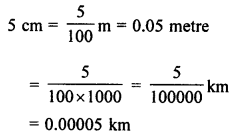Question 11.
Express in kg using decimals:
(i) 200 g
(ii) 3470 g
(iii) 4 kg 8 g
Solution:
(i) 200 g = $$\frac { 200 }{ 1000 }$$ = 0.2 kg
(ii) 3470 g = $$\frac { 3470 }{ 1000 }$$ = 3.470 kg
(iii) 4 kg 8 g = 4$$\frac { 8 }{ 1000 }$$ = 4.008 kg

Question 12.
(i) 5.765, 9.2, 3.08
(ii) 15.49, 8.3572, 0.903, 7.8
Solution:
(i) 5.765, 9.2, 3.08
Converting into like decimal (up to 3 places)
5.765, 9.200, 3.080
= 5.765 + 9.200 + 3.080
= 18.045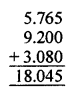(ii) 15.49, 8.3572, 0.903, 7.8
Converting into like decimals (up to 4 places)
= 15.4900 + 8.3572 + 0.9030, 7.8000
= 32.5502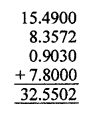Question 13.
Workout the following:
(i) 72.53 – 46.782
(ii) 18.376 – 5.43 – 8.8976
(iii) 28.5 – 9.708 – 6.234
(iv) 8.2 – 4.56 – 0.7912 + 2.67
Solution:
(i) 72.53 – 46.782
= 72.530 – 46.782
= 25.748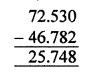(ii) 18.376 – 5.43 – 8.8976
= 18.3760 – 5.4300 – 8.8976 (Converting into like decimals)
= 18.3760 – 14.3276
= 4.0484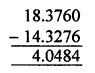(iii) 28.5 – 9.708 – 6.234
= 28.500 – 9.708 – 6.234
= 28.500 – 15.942
= 12.558(iv) 8.2 – 4.56 – 0.7912 + 2.67
= 8.2000 – 4.5600 – 0.7912 + 2.6700 (Converting into like decimals)
= 8.2000 + 2.6700 – 4.5600 – 0.7912
= 10.8700 – 5.3512
= 5.5188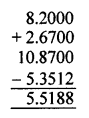Question 14.
(i) What number added to 3.56 gives 13.016?
(ii) What number should be subtracted from 30 to get 23.709?
(iii) What is the excess of 20.4 over 9.7403?
Solution:
(i) The required number = 13.016 – 3.56 = 13.016 – 3.560 = 9.456(ii) The required number = 30 – 23.709 = 30.000 – 23.709 = 6.291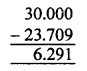(iii) The required number = 20.4 – 9.7403 = 20.4000 – 9.7403 = 10.6597